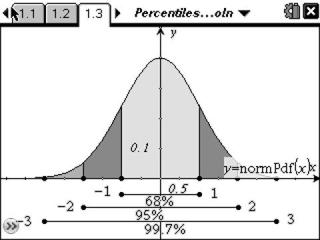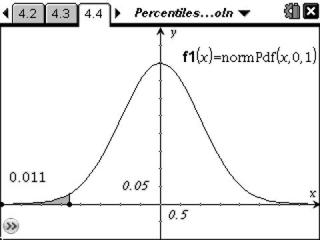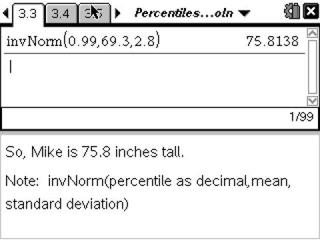# Activities

••• ##### Subject Area

• Math: Statistics: Normal Distributions

• ##### Author9-12

40 Minutes

• ##### Device
• TI-Nspire™ Navigator™
• TI-Nspire™
• TI-Nspire™ CAS
• ##### Software

TI-Nspire™
TI-Nspire™ CAS

2.0

• ##### Report an Issue

Percentiles & Z-Scores#### Activity Overview

Students explore percentiles and z-scores of normal distributions by graphing and calculating.

#### Key Steps

•The activity begins with a review of the properties of normal curves along with a visual representation of the corresponding percentages. The worksheet then introduces z-scores and percentiles.

•Students are given a range of x-values and asked to find the probability (or percent) of data that fall within the interval.

•In the last two parts, students are to find the corresponding x-value given a percentile and then given a z-score and they are asked to find the corresponding percentile and x-value.